GetFEM  5.4.2
bgeot::geometric_trans Class Referenceabstract

Description of a geometric transformation between a reference element and a real element. More...

#include <bgeot_geometric_trans.h>

Inherits dal::static_stored_object.

Inherited by bgeot::igeometric_trans< FUNC >, and bgeot::torus_geom_trans.

## Public Member Functions

dim_type dim () const
Dimension of the reference element.

bool is_linear () const
True if the transformation is linear (affine in fact).

size_type nb_points () const
Number of geometric nodes.

pconvex_ref convex_ref () const
Pointer on the convex of reference.

pconvex_structure structure () const
Structure of the reference element.

pconvex_structure basic_structure () const
Basic structure of the reference element.

virtual void poly_vector_val (const base_node &pt, base_vector &val) const =0
Gives the value of the functions vector at a certain point.

virtual void poly_vector_val (const base_node &pt, const convex_ind_ct &ind_ct, base_vector &val) const =0
Gives the value of a subgroup of the functions vector at a certain point.

virtual void poly_vector_grad (const base_node &pt, base_matrix &val) const =0
Gives the gradient of the functions vector at a certain point.

virtual void poly_vector_grad (const base_node &pt, const convex_ind_ct &ind_ct, base_matrix &val) const =0
Gives the gradient of a subgroup of the functions vector at a certain point.

virtual void poly_vector_hess (const base_node &pt, base_matrix &val) const =0
Gives the hessian of the functions vector at a certain point.

virtual void compute_K_matrix (const base_matrix &G, const base_matrix &pc, base_matrix &K) const
compute K matrix from multiplication of G with gradient

size_type nb_vertices () const
Gives the number of vertices.

const std::vector< size_type > & vertices () const
Gives the indices of vertices between the nodes.

const stored_point_tabgeometric_nodes () const
Gives the array of geometric nodes (on reference convex)

pstored_point_tab pgeometric_nodes () const
Gives the array of geometric nodes (on reference convex)

const std::vector< base_small_vector > & normals () const
Gives the array of the normals to faces (on reference convex)

template<class CONT >
base_node transform (const base_node &pt, const CONT &PTAB) const
Apply the geometric transformation to point pt, PTAB contains the points of the real convex.

## Detailed Description

Description of a geometric transformation between a reference element and a real element.

Geometric nodes and vector of polynomials. This class is not to be manipulate by itself. Use bgeot::pgeometric_trans and the functions written to produce the basic geometric transformations.

### Description of the geometry

Let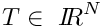be a real element and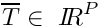be a reference element, with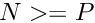.

The geometric nodes of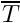are the points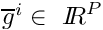, for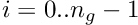, and the corresponding (via the geometric transformation) nodes of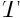are the points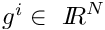.

### Geometric transformation

The geometric transformation is the application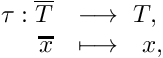which should be a diffeomorphism betweenand. It is assumed that there exists a (generally polynomial) vector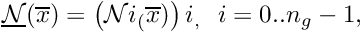defined onof size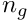, such that the transformation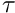can be written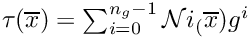.

Denoting by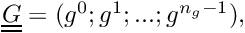The matrix in which each column is a geometric node of, the transformationcan be written as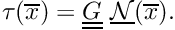The gradient of the transformation is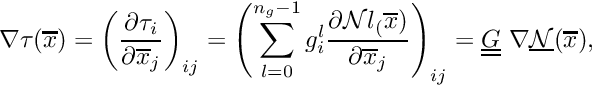Remark :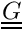is a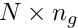matrix,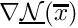is a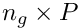matrix, and thus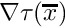is a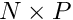matrix.

### Inverse transformation and pseudo-inverse

to do ...

Definition at line 105 of file bgeot_geometric_trans.h.

The documentation for this class was generated from the following files: